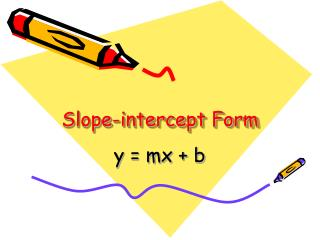DownloadDownload PresentationSlope-intercept Form

# Slope-intercept Form

Download Presentation## Slope-intercept Form

- - - - - - - - - - - - - - - - - - - - - - - - - - - E N D - - - - - - - - - - - - - - - - - - - - - - - - - - -
##### Presentation Transcript

1. Slope-intercept Form y = mx + b

2. An equation whose graph is a straight line is a linear equation. Since a function rule is an equation, a function can also be linear. m = slope b = y-intercept Y = mx + b (if you know the slope and where the line crosses the y-axis, use this form) Slope-intercept Form

3. For example in the equation;y = 3x + 6m = 3, so the slope is 3b = +6, so the y-intercept is +6 Let’s look at another: y = 4/5x -7 m = 4/5, so the slope is 4/5 b = -7, so the y-intercept is -7 Please note that in the slope-intercept formula; y = mx + b the “y” term is all by itself on the left side of the equation. That is very important!

4. WHY?If the “y” is not all by itself, then we must first use the rules of algebra to isolate the “y” term.For example in the equation: 2y = 8x + 10 You will notice that in order to get “y” all by itself we have to divide both sides by 2. After you have done that, the equation becomes: Y = 4x + 5 Only then can we determine the slope (4), and the y-intercept (+5)

5. OK…getting back to the lesson…Your job is to write the equation of a line after you are given the slope and y-intercept… Let’s try one… Given “m” (the slope remember!) = 2 And “b” (the y-intercept) = +9 All you have to do is plug those values into y = mx + b The equation becomes… y = 2x + 9

6. Let’s do a couple more to make sure you are expert at this. Given m = 2/3, b = -12, Write the equation of a line in slope-intercept form. Y = mx + b Y = 2/3x – 12 ************************* One last example… Given m = -5, b = -1 Write the equation of a line in slope-intercept form. Y = mx + b Y = -5x - 1

7. Given the slope and y-intercept, write the equation of a line in slope-intercept form. 1) m = 3, b = -14 2) m = -½, b = 4 3) m = -3, b = -7 4) m = 1/2 , b = 0 5) m = 2, b = 4 Slope-intercept form of an equation Y = mx + b

8. Using slope-intercept form to find slopes and y-interceptsThe graph at the right shows the equation of a line both in standard form and slope-intercept form.You must rewrite the equation 6x – 3y = 12 in slope-intercept to be able to identify the slope and y-intercept.

9. 3x – y = 14 -y = -3x + 14 -1 -1 -1 y = 3x – 14 or 3x – y = 14 3x = y + 14 3x – 14 = y x + 2y = 8 2y = -x + 8 2 2 2 y = -1x + 4 2 Using slope-intercept form to write equations, Rewrite the equation solving for y = to determine the slope and y-intercept.

10. Write each equation in slope-intercept form.Identify the slope and y-intercept. 2x + y = 10 -4x + y = 6 4x + 3y = 9 2x + y = 3 5y = 3x

11. 1) Find the slope. 4 – (-4)8 -1 – 3 -4 m = -2 2) Choose either point and substitute. Solve for b. y = mx + b (3, -4) -4 = (-2)(3) + b -4 = -6 + b 2 = b Substitute m and b in equation. Y = mx + b Y = -2x + 2 Write the equation of a line in slope-intercept form that passes through points (3, -4) and (-1, 4). Do you remember the slope formula?

12. Write the equation of the line in slope-intercept form that passes through each pair of points. • (-1, -6) and (2, 6) • (0, 5) and (3, 1) • (3, 5) and (6, 6) • (0, -7) and (4, 25) • (-1, 1) and (3, -3)